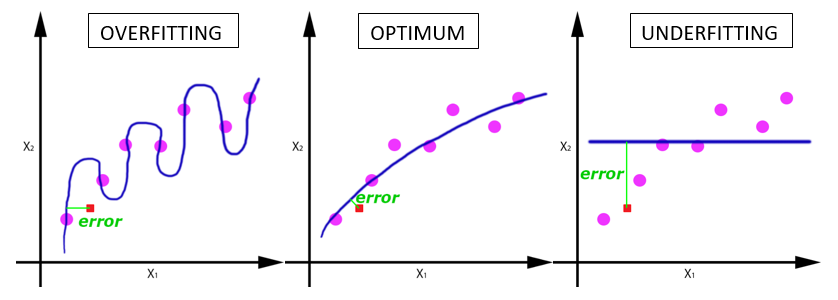/  Deep Learning Interview questions and answers   /  What do you mean by Epoch, Batch, Iterations in a Neural Network?## What do you mean by Epoch, Batch, Iterations in a Neural Network?

Ans:

Epoch

In real time we have very large datasets which we cannot feed to the computer all at once. Which means the entire Dataset is to be divided in order to feed to the Neural Network. Epoch is when the complete dataset is passed forward and backward through the Neural Network only once.

Batch

Epoch is too big to feed the computer at once. Hence, we need to divide them into several parts which are called as Batches. The dataset is passed to the same Neural Network multiple times. One Epoch in the Neural Network leads to underfitting of the curve, to avoid this problem we have to increase the number of Epochs. As the number of Epochs increases, a greater number of times the weights are changed in Neural Network and the curve goes from under fitting to optimal fitting to over fitting.  We cannot specify the value for number of Epochs, it is related to how diverse the dataset is. Batch size is total number of training samples present in one Batch. Batch size is different from Number of Batches.

Iterations

Iterations is the number of batches needed or required to complete one Epoch. Number of Batches is equal to number of iterations for one Epoch.